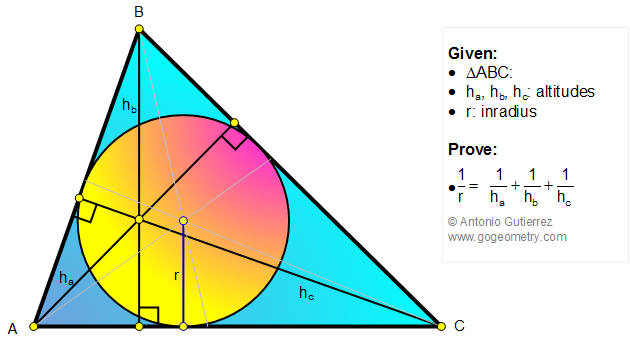# Geometry Problem 764: Triangle, Inradius, Altitudes, Harmonic Mean. Level: High School, College.

 The figure shows a triangle ABC with the inradius r and the altitudes ha, hb, hc. Prove that the inradius is one-third the harmonic mean of the altitudes, therefore, $$\dfrac{1}{r}=\dfrac{1}{h_{a}}+\dfrac{1}{h_{b}}+\dfrac{1}{h_{c}}$$Home | Search | Geometry | Problems | All Problems | Open Problems | Visual Index | 761-770 | Triangles | Altitude | Inradius | Harmonic Mean | Problem 1056 | Problem 1071 | Problem 195 | Email | Solution / comment | by Antonio Gutierrez